# Chapter 8 Solutions Characteristics of solutions Solutions are

• Slides: 53Chapter 8 Solutions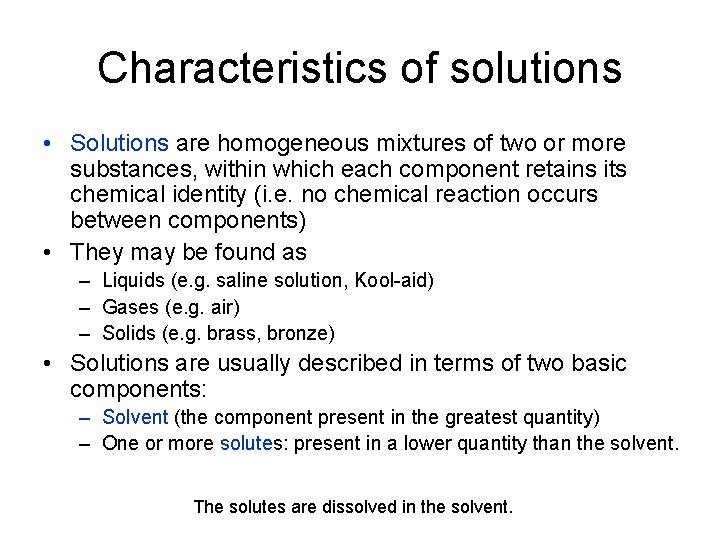Characteristics of solutions • Solutions are homogeneous mixtures of two or more substances, within which each component retains its chemical identity (i. e. no chemical reaction occurs between components) • They may be found as – Liquids (e. g. saline solution, Kool-aid) – Gases (e. g. air) – Solids (e. g. brass, bronze) • Solutions are usually described in terms of two basic components: – Solvent (the component present in the greatest quantity) – One or more solutes: present in a lower quantity than the solvent. The solutes are dissolved in the solvent.Characteristics of solutions 1. Solutions contain two or more components (solvent and one or more solutes) 2. Solutions can have variable composition (can vary the solute(s)-solvent ratio) 3. The properties of a solution will change as the solute(s)solvent ratio is changed 4. Dissolved solutes are present as individual particles that “mingle” with the solvent particles (through intermolecular forces of attraction) 5. Solutes are uniformly distributed within the solution and do not settle out over time 6. Solvents can generally be evaporated by physical means to obtain the solvent in its original form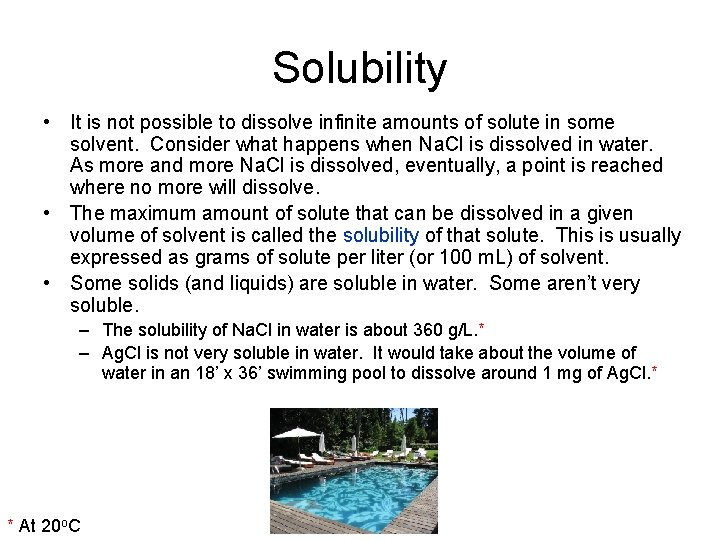Solubility • It is not possible to dissolve infinite amounts of solute in some solvent. Consider what happens when Na. Cl is dissolved in water. As more and more Na. Cl is dissolved, eventually, a point is reached where no more will dissolve. • The maximum amount of solute that can be dissolved in a given volume of solvent is called the solubility of that solute. This is usually expressed as grams of solute per liter (or 100 m. L) of solvent. • Some solids (and liquids) are soluble in water. Some aren’t very soluble. – The solubility of Na. Cl in water is about 360 g/L. * – Ag. Cl is not very soluble in water. It would take about the volume of water in an 18’ x 36’ swimming pool to dissolve around 1 mg of Ag. Cl. * * At 20 o. C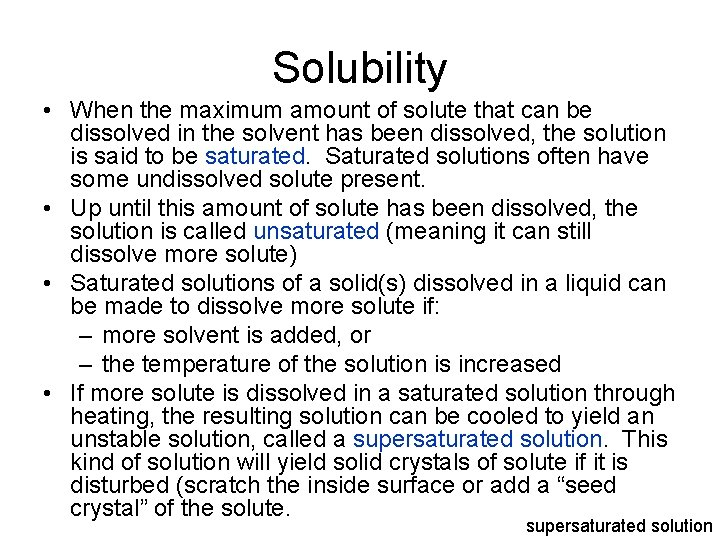Solubility • When the maximum amount of solute that can be dissolved in the solvent has been dissolved, the solution is said to be saturated. Saturated solutions often have some undissolved solute present. • Up until this amount of solute has been dissolved, the solution is called unsaturated (meaning it can still dissolve more solute) • Saturated solutions of a solid(s) dissolved in a liquid can be made to dissolve more solute if: – more solvent is added, or – the temperature of the solution is increased • If more solute is dissolved in a saturated solution through heating, the resulting solution can be cooled to yield an unstable solution, called a supersaturated solution. This kind of solution will yield solid crystals of solute if it is disturbed (scratch the inside surface or add a “seed crystal” of the solute. supersaturated solutionSolubility • The solubility of a solid solute in water can be increased by increasing the temperature of the mixture. • If the solution consists of a gas dissolved in a liquid, the gas’s solubility will decrease with increasing temperature. • The solubility of a gas in a liquid can be increased by increasing the partial pressure of the gas above the liquid: – Henry’s Law: the solubility of a gas in a liquid is directly proportional to the partial pressure of the gas above the liquidSolubility • Solutions in which the solvent is water are called aqueous solutions (as we talked about in chapter 6 – in a balanced chemical equation, these species are indicated with (aq) after them) • Solutions for which the solvent is not water are called non-aqueous solutions (for example, some solutions employ ethanol as a solvent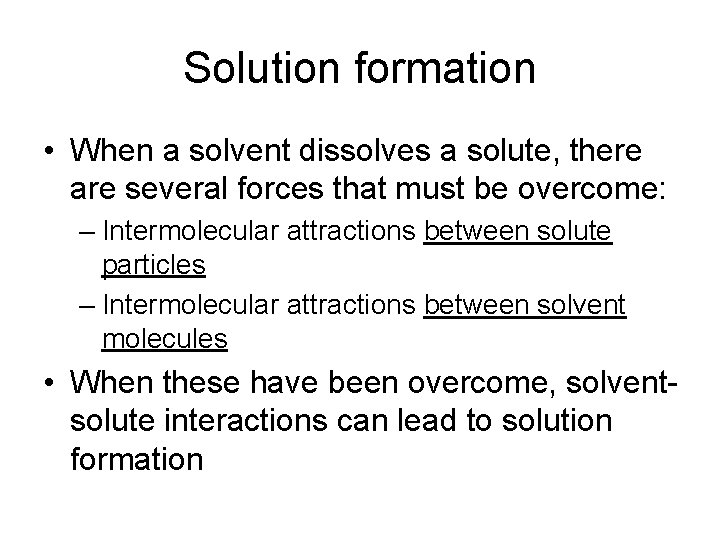Solution formation • When a solvent dissolves a solute, there are several forces that must be overcome: – Intermolecular attractions between solute particles – Intermolecular attractions between solvent molecules • When these have been overcome, solventsolute interactions can lead to solution formationSolution formation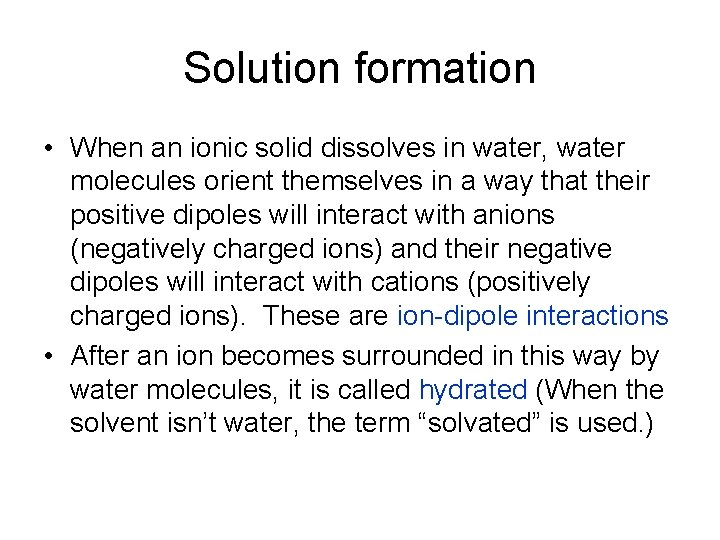Solution formation • When an ionic solid dissolves in water, water molecules orient themselves in a way that their positive dipoles will interact with anions (negatively charged ions) and their negative dipoles will interact with cations (positively charged ions). These are ion-dipole interactions • After an ion becomes surrounded in this way by water molecules, it is called hydrated (When the solvent isn’t water, the term “solvated” is used. )Factors affecting the rate of solution formation 1. The state of subdivision of the solute (the greater the exposed surface area of the solute, the faster it will dissolve) 2. The degree of agitation during solution preparation (solutes will dissolve faster when the mixture is stirred, as particles are dispersed, increasing the likelihood of solutesolvent interactions) 3. The temperature of the components (solution formation is faster as the temperature is raised, since the components will possess more kinetic energy)Solubility rules • As mentioned earlier, Ag. Cl is not very water-soluble. • In general, as the polarity of the solvent and solute become less alike, the less favorable will be interactions between the solute and the solvent. • Substances of like polarity tend to be more soluble in each other than substances whose polarities differ • This rule is very good at predicting solubilities of gases and liquids in liquid solvents. For solid-liquid mixtures, the results for ionic solids are not always in agreement, because: – Ion-ion interactions are influenced by both ion size and chargeSolubility rules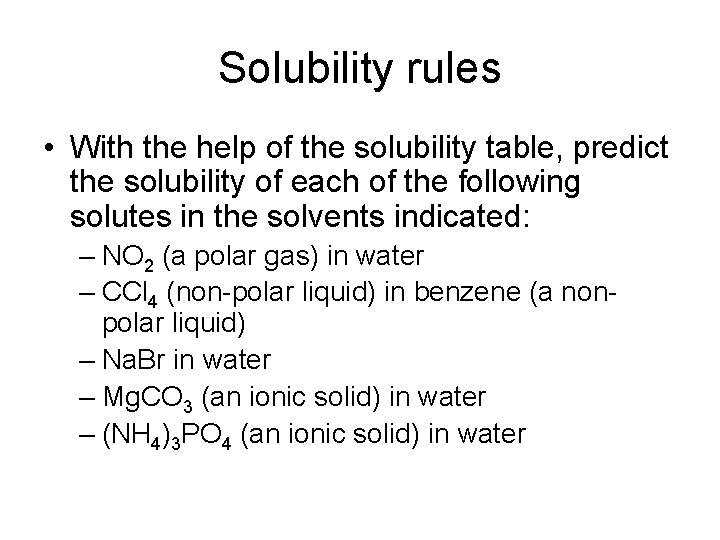Solubility rules • With the help of the solubility table, predict the solubility of each of the following solutes in the solvents indicated: – NO 2 (a polar gas) in water – CCl 4 (non-polar liquid) in benzene (a nonpolar liquid) – Na. Br in water – Mg. CO 3 (an ionic solid) in water – (NH 4)3 PO 4 (an ionic solid) in waterSolution concentration units • The composition of a solution is expressed in terms of its concentration. Concentration indicates the amount of solute that is dissolved in a given quanitity of solution. • Concentration is commonly expressed in one of two ways: – Percent concentration – MolaritySolution concentration units Percentage concentrations • Three different ways that percent concentrations are expressed are as follows: – Percent by mass - or mass-mass percent, %(m/m) – Percent by volume- or volume-volume percent, %(v/v) – Mass-volume percent, %(m/v)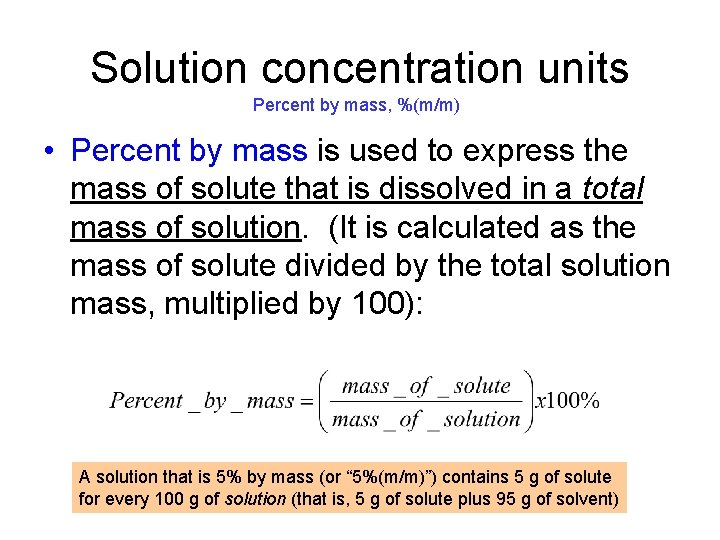Solution concentration units Percent by mass, %(m/m) • Percent by mass is used to express the mass of solute that is dissolved in a total mass of solution. (It is calculated as the mass of solute divided by the total solution mass, multiplied by 100): A solution that is 5% by mass (or “ 5%(m/m)”) contains 5 g of solute for every 100 g of solution (that is, 5 g of solute plus 95 g of solvent)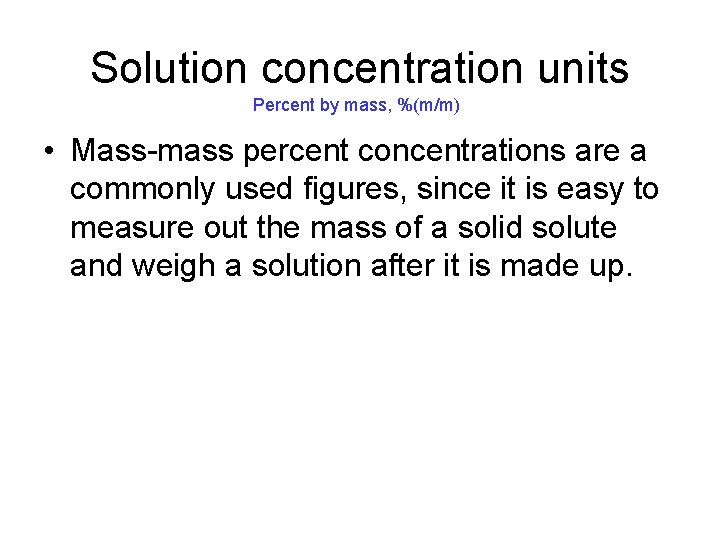Solution concentration units Percent by mass, %(m/m) • Mass-mass percent concentrations are a commonly used figures, since it is easy to measure out the mass of a solid solute and weigh a solution after it is made up.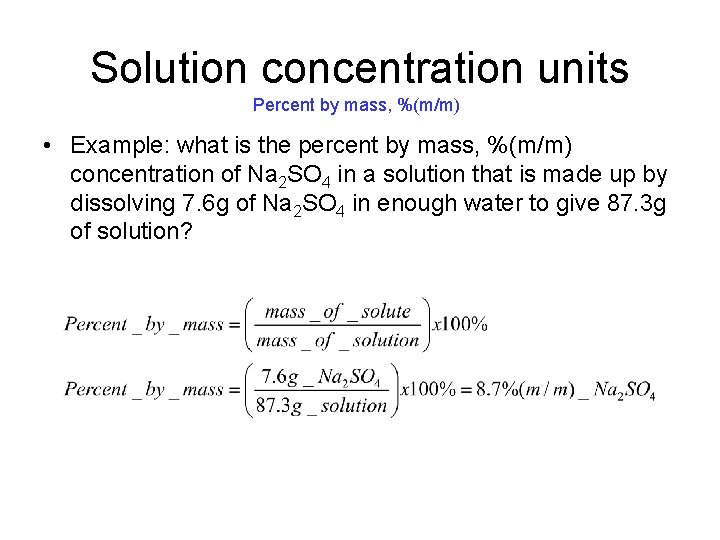Solution concentration units Percent by mass, %(m/m) • Example: what is the percent by mass, %(m/m) concentration of Na 2 SO 4 in a solution that is made up by dissolving 7. 6 g of Na 2 SO 4 in enough water to give 87. 3 g of solution?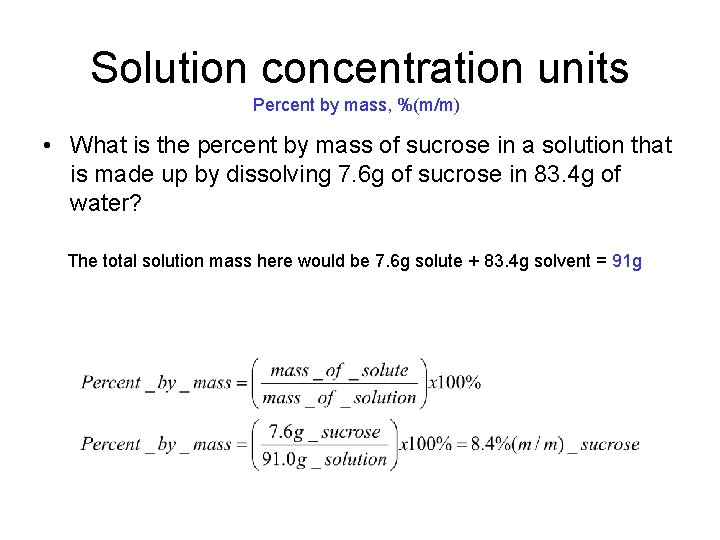Solution concentration units Percent by mass, %(m/m) • What is the percent by mass of sucrose in a solution that is made up by dissolving 7. 6 g of sucrose in 83. 4 g of water? The total solution mass here would be 7. 6 g solute + 83. 4 g solvent = 91 g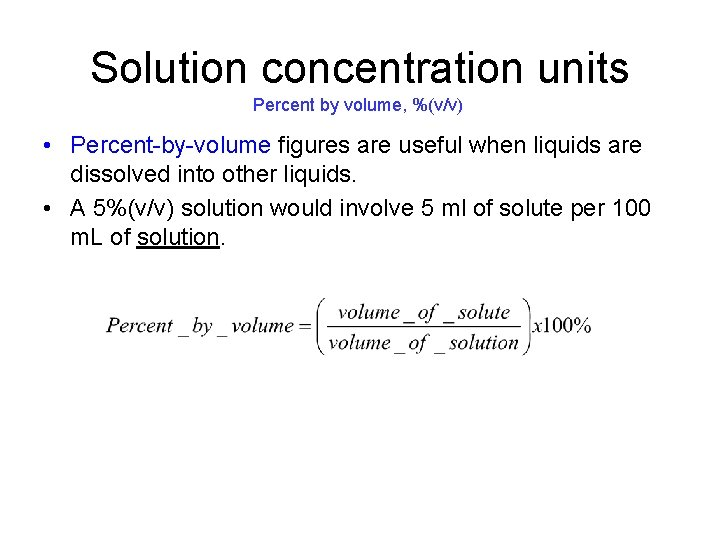Solution concentration units Percent by volume, %(v/v) • Percent-by-volume figures are useful when liquids are dissolved into other liquids. • A 5%(v/v) solution would involve 5 ml of solute per 100 m. L of solution.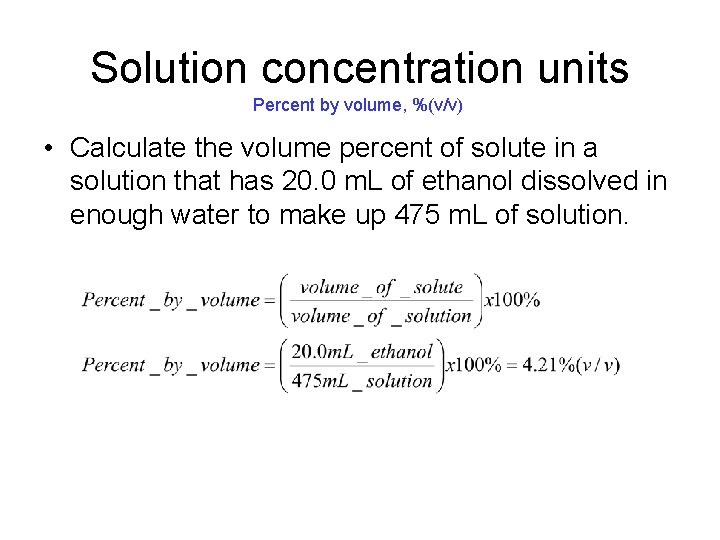Solution concentration units Percent by volume, %(v/v) • Calculate the volume percent of solute in a solution that has 20. 0 m. L of ethanol dissolved in enough water to make up 475 m. L of solution.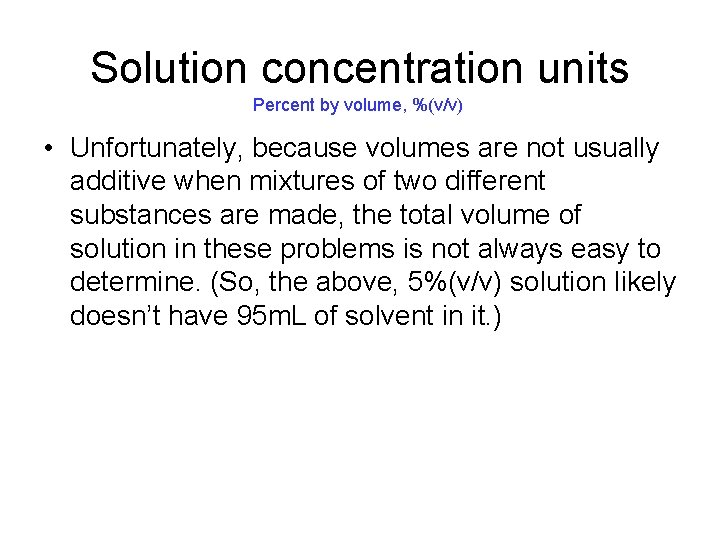Solution concentration units Percent by volume, %(v/v) • Unfortunately, because volumes are not usually additive when mixtures of two different substances are made, the total volume of solution in these problems is not always easy to determine. (So, the above, 5%(v/v) solution likely doesn’t have 95 m. L of solvent in it. )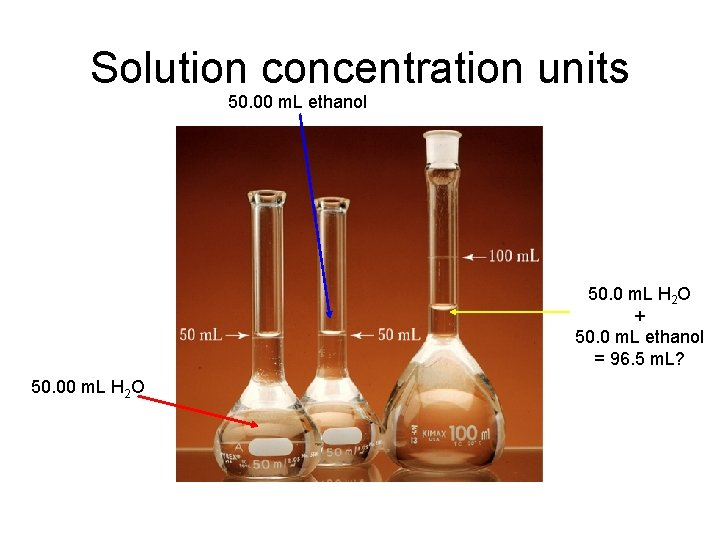Solution concentration units 50. 00 m. L ethanol 50. 0 m. L H 2 O + 50. 0 m. L ethanol = 96. 5 m. L? 50. 00 m. L H 2 O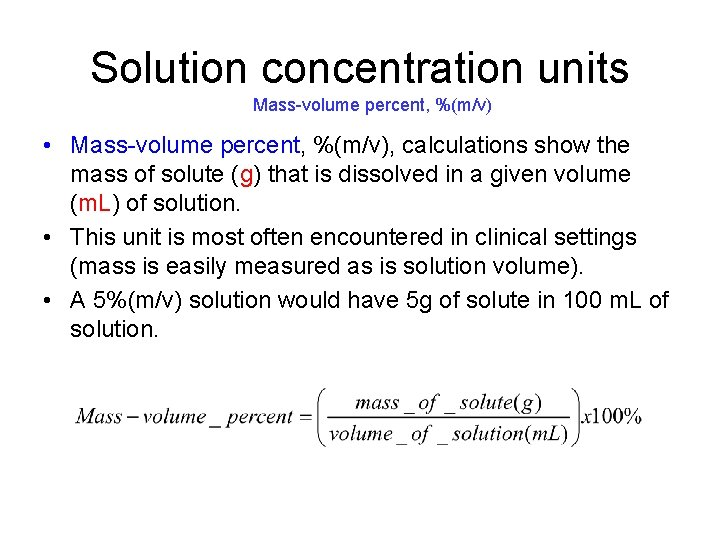Solution concentration units Mass-volume percent, %(m/v) • Mass-volume percent, %(m/v), calculations show the mass of solute (g) that is dissolved in a given volume (m. L) of solution. • This unit is most often encountered in clinical settings (mass is easily measured as is solution volume). • A 5%(m/v) solution would have 5 g of solute in 100 m. L of solution.Solution concentration units Mass-volume percent, %(m/v) • How many grams of glucose must be added to prepare 500. 0 m. L of a 4. 50%(m/v) glucose-water solution?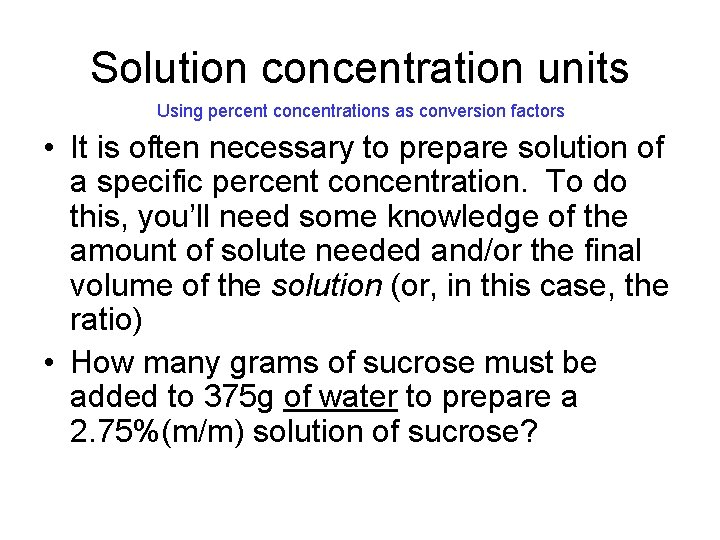Solution concentration units Using percent concentrations as conversion factors • It is often necessary to prepare solution of a specific percent concentration. To do this, you’ll need some knowledge of the amount of solute needed and/or the final volume of the solution (or, in this case, the ratio) • How many grams of sucrose must be added to 375 g of water to prepare a 2. 75%(m/m) solution of sucrose?Solution concentration units Using percent concentrations as conversion factors • How many grams of sucrose must be added to 375 g of water to prepare a 2. 75%(m/m) solution of sucrose? Don’t know the solution mass here. If we did, we could just plug things into: …and then solve for the mass of sucrose. Since we don’t know the total solution mass, we have to determine the mass of water that is in a 2. 75%(m/m) solution. Do this assuming 100 g solution: 100 g of solution – 2. 75 g of sucrose = 97. 25 g of waterSolution concentration units Using percent concentrations as conversion factors • Now, we can make a conversion factor to determine the mass of sucrose needed to be added to the 375 g of water The last calculation tells us that each 2. 75 g of sucrose needs to be added to 97. 5 g of water to make up this solution: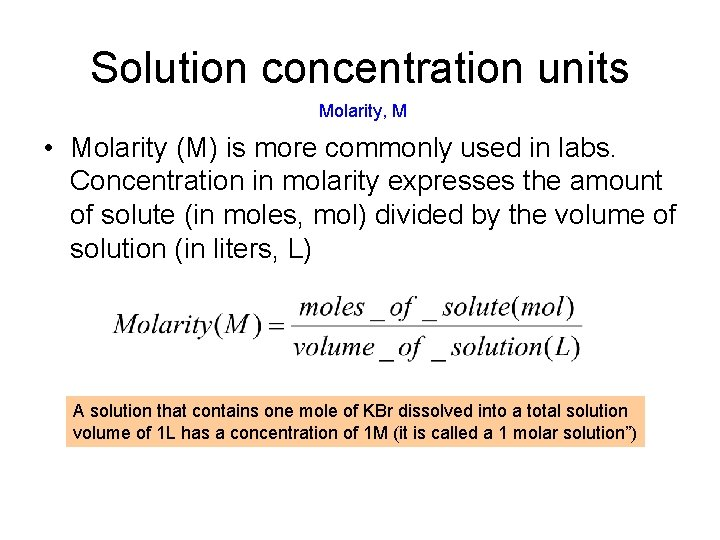Solution concentration units Molarity, M • Molarity (M) is more commonly used in labs. Concentration in molarity expresses the amount of solute (in moles, mol) divided by the volume of solution (in liters, L) A solution that contains one mole of KBr dissolved into a total solution volume of 1 L has a concentration of 1 M (it is called a 1 molar solution”)Solution concentration units Molarity, M • In order to find the concentration of a solution in molarity, the solution volume needs to be known and the number of moles of solute as well. This may involve a prior calculation if the mass of solute is given instead.Solution concentration units Molarity, M Q: What is the concentration (M) of a solution that is made up by dissolving 12. 5 g of Na. Cl in enough water to make up 175 m. L of solution? A: First, how many moles of Na. Cl is 12. 5 g of Na. Cl? . . . need the molar mass for Na. Cl From the periodic table: Na+Cl 22. 99 + 35. 45 = 58. 44 1 mol Na. Cl = 58. 44 g Na. Cl need volume in liters!!Solution concentration units Molarity, M • How many grams of sucrose (C 12 H 22 O 11) are present in 185 m. L of a 2. 50 M solution of sucrose? Can’t determine this directly from the concentration (it gives moles of sucrose per liter of solution) Could determine the number of moles of sucrose in 185 m. L of the solution, then multiply that quantity by the molar mass for sucrose to get mass Molar mass: 1 mol C 12 H 22 O 11 = 342. 34 g C 12 H 22 O 11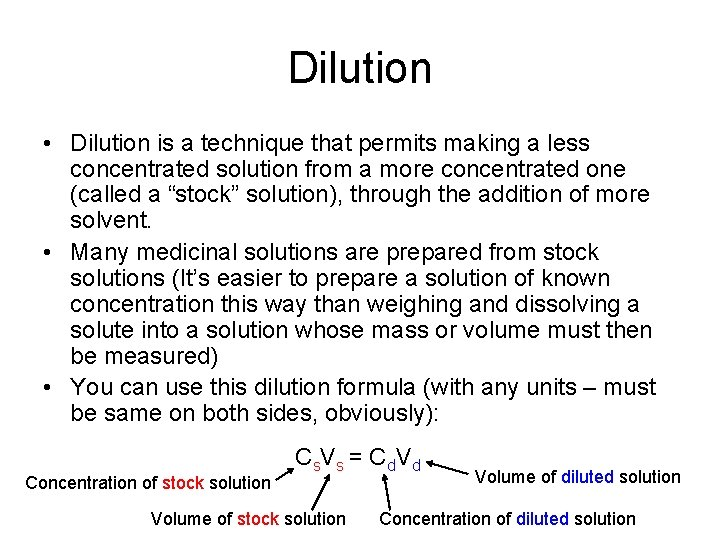Dilution • Dilution is a technique that permits making a less concentrated solution from a more concentrated one (called a “stock” solution), through the addition of more solvent. • Many medicinal solutions are prepared from stock solutions (It’s easier to prepare a solution of known concentration this way than weighing and dissolving a solute into a solution whose mass or volume must then be measured) • You can use this dilution formula (with any units – must be same on both sides, obviously): Concentration of stock solution C s. V s = C d Volume of stock solution Volume of diluted solution Concentration of diluted solution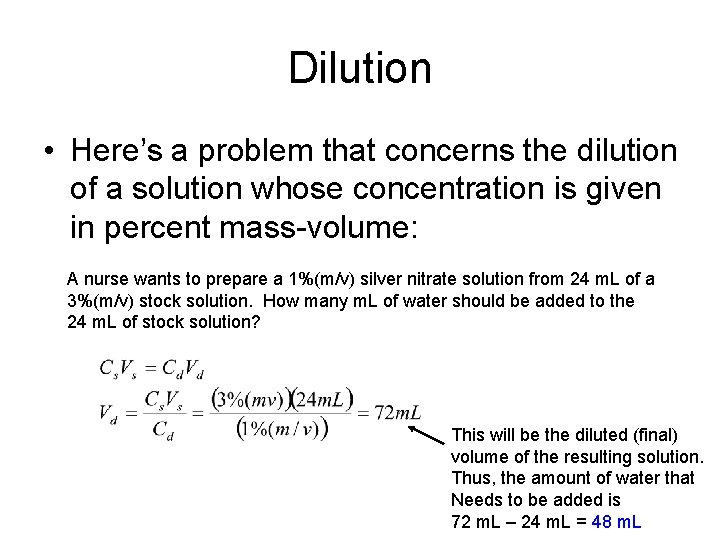Dilution • Here’s a problem that concerns the dilution of a solution whose concentration is given in percent mass-volume: A nurse wants to prepare a 1%(m/v) silver nitrate solution from 24 m. L of a 3%(m/v) stock solution. How many m. L of water should be added to the 24 m. L of stock solution? This will be the diluted (final) volume of the resulting solution. Thus, the amount of water that Needs to be added is 72 m. L – 24 m. L = 48 m. LDilution • What is the molarity of the solution prepared by diluting 65 m. L of 0. 95 M Na 2 SO 4 solution to a final volume of 135 m. L?Colligative and colloidal properties • Solutions are homogeneous mixtures made by dissolving a solute into a solvent • Dispersions are homogeneous mixtures that contain dispersed particles that are intermediate is size between those of a true solution and those of an ordinary heterogeneous mixture • The terms solute and solvent don’t apply for colloidal dispersions. Instead, the terms dispersed phase (like a solute) and dispersing medium (like a solvent) are usedColligative and colloidal properties • For colloidal dispersions, the situation is similar. Particles are very small, so small that – They don’t settle out over time under the influence of gravity – They aren’t able to be detected by the naked eye – They can’t be filtered using filter paper that has relatively large pores • On the other hand, the presence of the dispersed phase causes the path of a light beam to be discernible Light beam is scattered by the dispersed phase. Called Tyndall scattering Tyndall effect: the light-scattering phenomenon that causes the path of a beam of light through a colloidal dispersion to be visible.Colligative and colloidal properties • • • In a dispersion, dissolved particles have sizes of the order of 10 -7 cm to 10 -5 cm (nm to hundreds of nm range) Dissolved ions in solutions, by comparison, have diameters less than 10 -7 cm In mixtures that have particles of diameters that are greater than 105 cm, the particles tend to settle out over time. Such mixtures are called suspensions, and a good example of a suspension is muddy water (though another example would be salad dressing)Colligative properties of solutions • When a solute is added to a solvent, the physical properties of the resulting solution are different that the pure solvent. • Colligative properties are physical properties of solutions that depend on the number (concentration) of solute particles (molecules or ions) in a given quantity of solvent, and not on their chemical identities. • Colligative properties of solutions: – – Vapor pressure Boiling point elevation Freezing point depression Osmotic pressure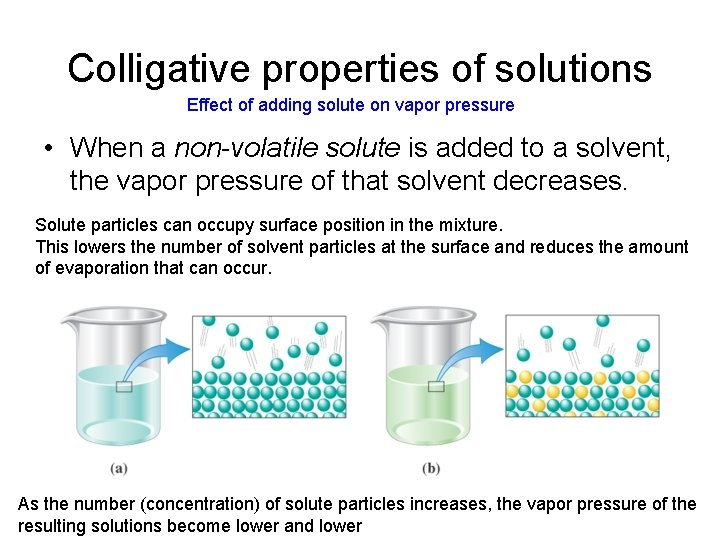Colligative properties of solutions Effect of adding solute on vapor pressure • When a non-volatile solute is added to a solvent, the vapor pressure of that solvent decreases. Solute particles can occupy surface position in the mixture. This lowers the number of solvent particles at the surface and reduces the amount of evaporation that can occur. As the number (concentration) of solute particles increases, the vapor pressure of the resulting solutions become lower and lower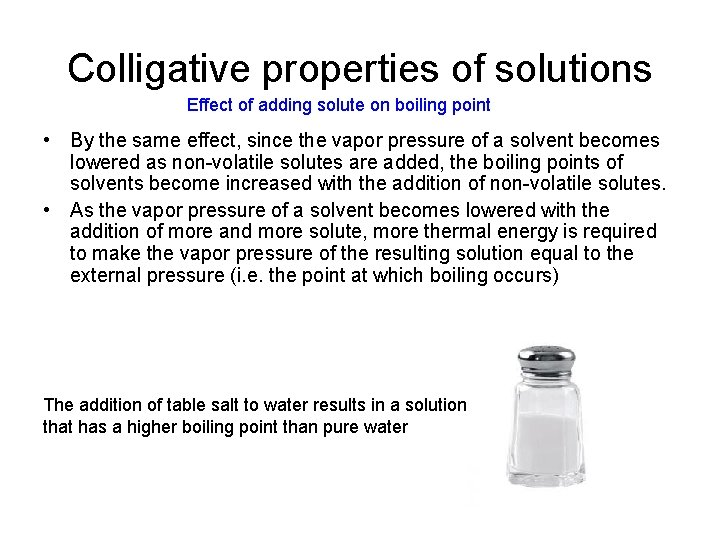Colligative properties of solutions Effect of adding solute on boiling point • By the same effect, since the vapor pressure of a solvent becomes lowered as non-volatile solutes are added, the boiling points of solvents become increased with the addition of non-volatile solutes. • As the vapor pressure of a solvent becomes lowered with the addition of more and more solute, more thermal energy is required to make the vapor pressure of the resulting solution equal to the external pressure (i. e. the point at which boiling occurs) The addition of table salt to water results in a solution that has a higher boiling point than pure waterColligative properties of solutions Effect of adding solute on freezing point • The addition of solutes to a pure solvent also has the effect of lowering the solvent freezing point. • The added solute particles interfere with the solvent molecules’ ability to form regular, crystalline structures. • Consequently, lower temperatures must be used to drive the freezing process.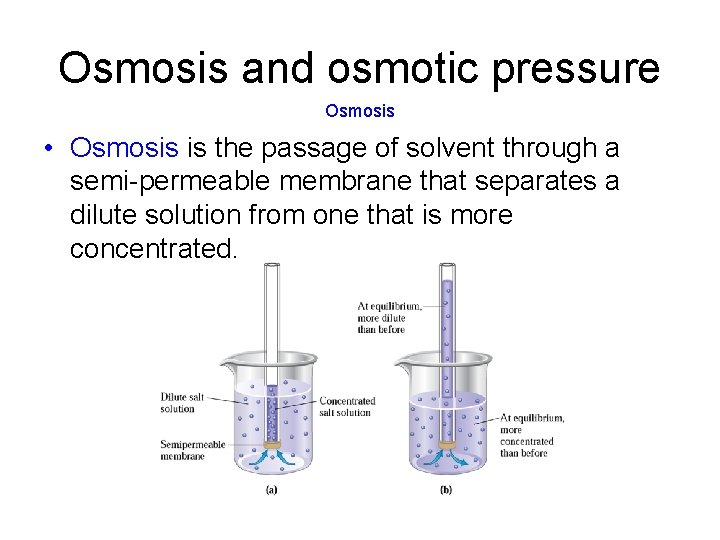Osmosis and osmotic pressure Osmosis • Osmosis is the passage of solvent through a semi-permeable membrane that separates a dilute solution from one that is more concentrated.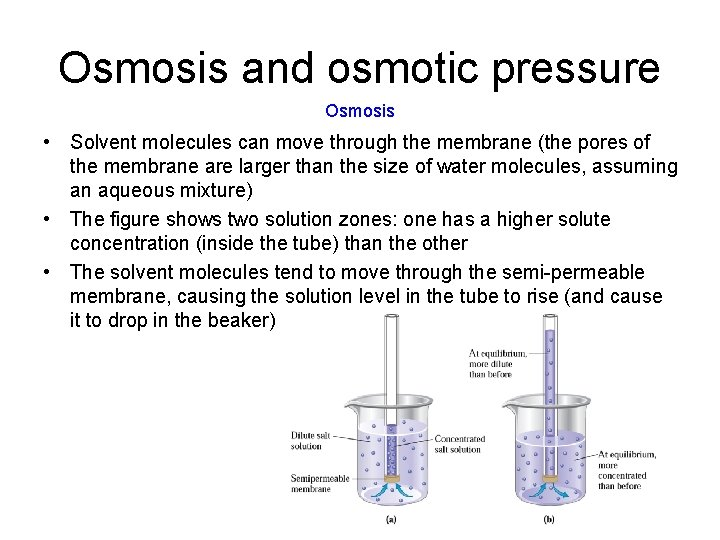Osmosis and osmotic pressure Osmosis • Solvent molecules can move through the membrane (the pores of the membrane are larger than the size of water molecules, assuming an aqueous mixture) • The figure shows two solution zones: one has a higher solute concentration (inside the tube) than the other • The solvent molecules tend to move through the semi-permeable membrane, causing the solution level in the tube to rise (and cause it to drop in the beaker)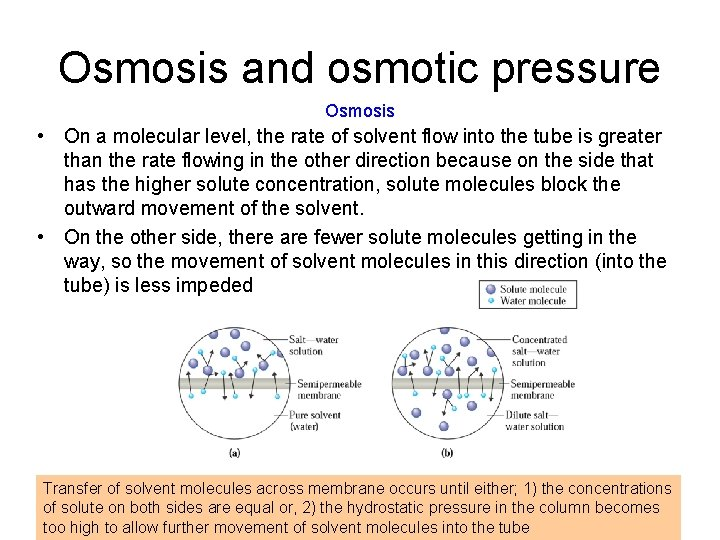Osmosis and osmotic pressure Osmosis • On a molecular level, the rate of solvent flow into the tube is greater than the rate flowing in the other direction because on the side that has the higher solute concentration, solute molecules block the outward movement of the solvent. • On the other side, there are fewer solute molecules getting in the way, so the movement of solvent molecules in this direction (into the tube) is less impeded Transfer of solvent molecules across membrane occurs until either; 1) the concentrations of solute on both sides are equal or, 2) the hydrostatic pressure in the column becomes too high to allow further movement of solvent molecules into the tube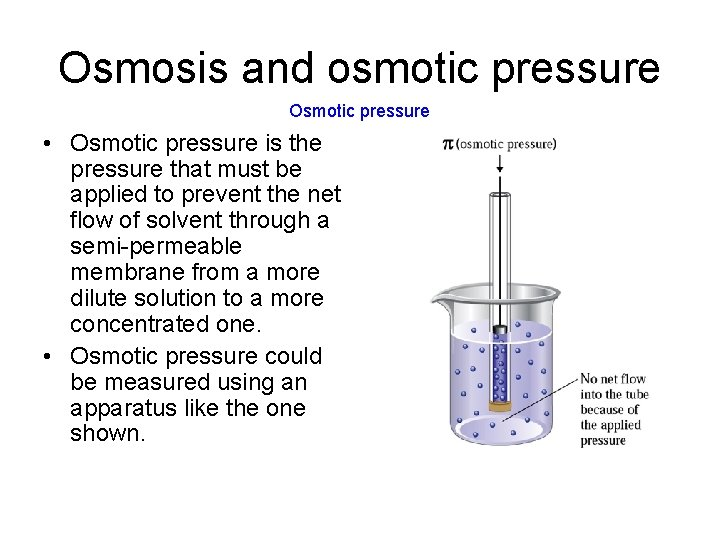Osmosis and osmotic pressure Osmotic pressure • Osmotic pressure is the pressure that must be applied to prevent the net flow of solvent through a semi-permeable membrane from a more dilute solution to a more concentrated one. • Osmotic pressure could be measured using an apparatus like the one shown.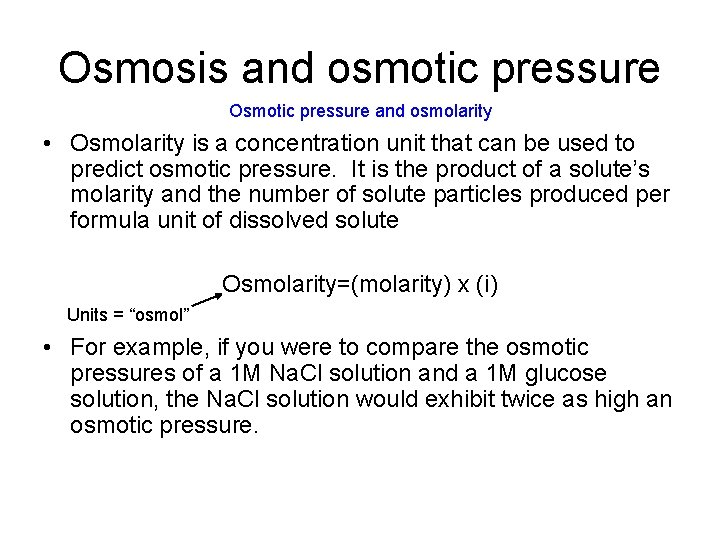Osmosis and osmotic pressure Osmotic pressure and osmolarity • Osmolarity is a concentration unit that can be used to predict osmotic pressure. It is the product of a solute’s molarity and the number of solute particles produced per formula unit of dissolved solute Osmolarity=(molarity) x (i) Units = “osmol” • For example, if you were to compare the osmotic pressures of a 1 M Na. Cl solution and a 1 M glucose solution, the Na. Cl solution would exhibit twice as high an osmotic pressure.Osmosis and osmotic pressure Hypotonic, hypertonic, and isotonic solutions • The terms, hypotonic, hypertonic, and isotonic solutions refer to osmotic-type phenomena that occur in the human body • Usually these terms are referenced to the osmotic pressure of cells (e. g. , red blood cells) • Hypotonic solutions are solutions that exert a lower osmotic pressure than the solution contained inside cells • Hypertonic solutions exert a higher osmotic pressure than cellular solutions • Isotonic solutions have the same osmotic pressure as cellular solutionsOsmosis and osmotic pressure • When red blood cells are placed in hypotonic solutions (e. g. pure water), water moves through the cell membrane into the cell and results in an increase in cell volume, which results in the cells finally rupturing. The process is known as hemolysis. HemolysisOsmosis and osmotic pressure • When red blood cells are placed an a hypertonic solution, the opposite effect results. Na. Cl solutions having greater than 0. 9%(m/v) Na. Cl are hypertonic. • Water leaves the cell and the cell structure is again adversely affected (“crenation”) CrenationOsmosis and osmotic pressure • Isotonic solutions exert the same osmotic pressure as the cell solution, so there is no net outward or inward migration of solvent molecules. • Red blood cells places in isotonic solutions (e. g. 5%(m/v) glucose) are stable. • Intravenous solutions will often use isotonic solutions for the introduction of nutrients to the body. in an isotonic solution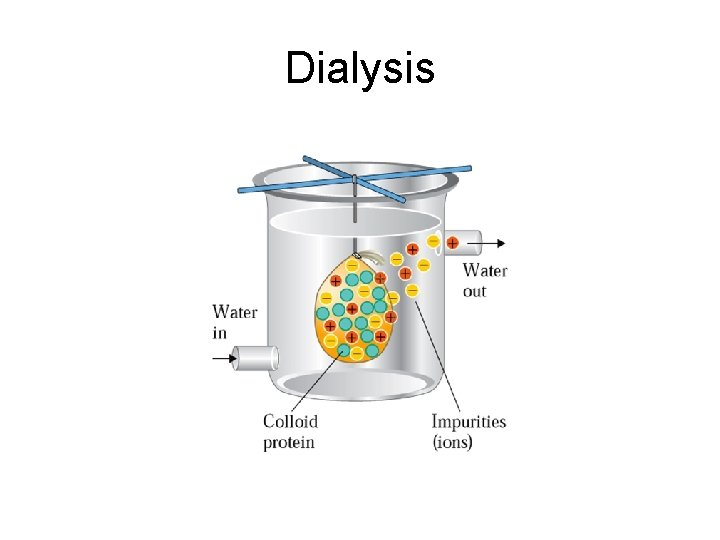Dialysis